# MCQ Questions for Class 7 Maths Chapter 7 Congruence of Triangles with Answers

We have compiled the NCERT MCQ Questions for Class 7 Maths Chapter 7 Congruence of Triangles with Answers Pdf free download covering the entire syllabus. Practice MCQ Questions for Class 7 Maths with Answers on a daily basis and score well in exams. Refer to the Congruence of Triangles Class 7 MCQs Questions with Answers here along with a detailed explanation.

## Congruence of Triangles Class 7 MCQs Questions with Answers

Choose the correct option.

Question 1.
For two given triangles ABC and PQR, how many matching are possible?
(a) 2
(b) 3
(c) 4
(d) 6

Question 2.
It ∆ABC ≅ ∆PQR then $$\bar{AB}$$ is equal to
(a) $$\bar{PQ}$$
(b) $$\bar{OR}$$
(c) $$\bar{RP}$$
(d) none of these

Answer: (a) $$\bar{PQ}$$

Question 3.
In the given figure, if AB = AC and BD = DC then ∠ADC =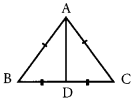(a) 45°
(b) 60°
(c) 125°
(d) 90°

Question 4.
By which congruence property, the two triangles given are congruent?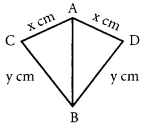(a) S.S.S Property
(b) R.H.S Property
(c) A. S.A Property
(d) S.A.S Property

Question 5.
If ∆ABC ≅ ∆PQR, then the value of ∠A is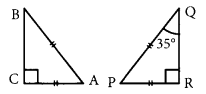(a) 35°
(b) 55°
(c) 90°
(d) 45°

Question 6.
Which of the following rules of congruency says that ∆ABC ≅ ∆PQR ?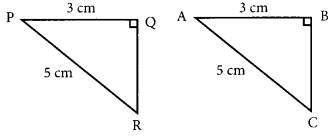(a) RHS
(b) SSS
(c) ASA
(d) SAS

Question 7.
Which of the following rules of congruency says that ∆ABC ≅ ∆PQR?(a) ASA
(b) SAS
(c) SSS
(d) RHS

Question 8.
Which congruence criterion do you use in the following?
Given EB = DB
AE = BC
∠A = ∠C = 90°
so ∆AEB ≅ ∆CDB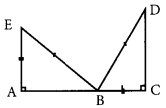(a) SSS
(b) SAS
(c) ASA
(d) RHS

Question 9.
Complete the congruence statement ∆BCA ≅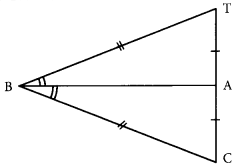(a) ∆BTA
(b) ∆BAT
(c) ∆ABT
(d) ∆ATB

Question 10.
Complete the congruence statement ∆QRS ≅ ?(a) ∆TQP
(b) ∆TPQ
(c) ∆QTP
(d) ∆QPT

Question 11.
Number of elements of a triangle is:
(a) 2
(b) 3
(c) 4
(d) 6

Question 12.
Two figures are said to be congruent, if they have exactly the same:
(a) length and width
(b) shape and size
(c) area
(d) perimeter

Fill in the blanks

Question 1.
If ∆ABC is congruent to ∆PQR such that ∠PQR = 125° then the measure of ∠ABC = …………………

Question 2.
In ∆ABC and ∆DEF, ∠B = ∠E, ∠B = ∠E and AC = DF then ∆ABC ………………… ∆DEF.

Question 3.
If ∆PQR ≅ ∆XYZ and XY = 9 m, YZ = 5 cm. then the length of PQ = ……………….

Question 4.
In ∆PQR, the included angle between QR and PR is ……………….

Question 5.
Two rectangles are congruent if they have the same …………….. and ………………..

Question 6.
If ∆ABC ≅ ∆DEF, then
(i) ∠A = ……………..
(ii) $$\bar{AB}$$ = ………………….

(i) ∠D
(ii) $$\bar{DE}$$

Question 7.
If ∆PQR ≅ ∆BAC, then
(i) ∠A = …………… [∠P/∠Q]
(ii) $$\bar{RQ}$$ = ………………. ($$\bar{CA}$$/$$\bar{AB}$$)

(i) ∠Q
(ii) $$\bar{CA}$$

Question 8.
If ∆XYZ ≅ ∆GDF, then
(i) Vertex X corresponds to vertex ……………
(ii) Side $$\bar{YZ}$$ = Side …………………

(i) G
(ii) $$\bar{DF}$$

Question 9.
If ∆PQR ≅ ∆OMN, then
(i) ∠P = ……………
(ii) $$\bar{RP}$$ = ……………..

(i) ∠O
(ii) $$\bar{NO}$$

Question 10.
In ∆PQR, the included side between ∠P and ∠Q is ……………….

Answer: $$\bar{PQ}$$

Question 11.
In ∆ABC, the included angle between $$\bar{AC}$$ and $$\bar{BC}$$ is ……………….

Question 12.
Two angles are said to be congruent, if they have ………………… [equal measures/equal sides]

Question 13.
Two plane figures are congruent, if they have …………………. [same shape/same shape and size]

Question 14.
Two squares are congruent if they have the same ……………..

Question 15.
Two rectangles are congruent if they have the same ……………….

Question 16.
When two angles and the included side of one triangle are respectively equal to two angles and the included side of another triangle. This is called the …………………….. congruence of triangles.